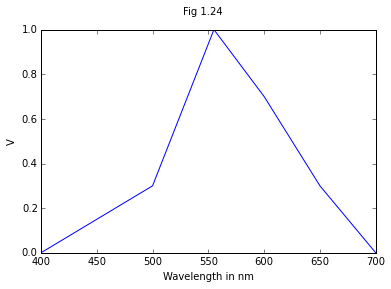Chapter Four : Display Devices¶

Example 4.1, Page Number 131¶

In :
from math import exp

k=0.025 #in Electron Volts
E=0.4 #in Electron Volts (The difference between Ec & Ed)
Q=(10**8) # A given Constant in per second

j=E/k
O=Q*exp(-j) #Where O is the required probability
O=round(O,2)
Q1=1/O
Q1=round(Q1,2)
print "The probability of Escape per second of the trapped Electron "+str(O)+" /s"
print "The Luminescence Lifetime is "+str(Q1)+" seconds"
The probability of Escape per second of the trapped Electron 11.25 /s
The Luminescence Lifetime is 0.09 seconds

Example 4.2, Page Number 152¶

In :
from math import degrees
from math import asin

n1=3.6  #For a Given GaAs/Air Interface
n2=1    #For Air

#Using Equation 4.14
n3=n1-n2
n4=n1+n2
n6=(n3/n4)**2
n5=(n2/n1)**2

F=0.25*(n5)*(1-n6) #Where F is the Fractional Transmission for Isotropic Radiation Originating
F=round(F,3)

theta=degrees(asin(1/n1))  #Critical Angle in Degrees
theta=round(theta,0)

print "The Fractional Tranmission for Isotropic Radiation originating inside GaAs is "+str(F)
print "The Critical Angle which might explain the Low efficiency for the interface is "+str(theta)+" Degrees"
The Fractional Tranmission for Isotropic Radiation originating inside GaAs is 0.013
The Critical Angle which might explain the Low efficiency for the interface is 16.0 Degrees

Example 4.3, Page Number 158¶

In :
%matplotlib inline
import math
from matplotlib.pyplot import plot,suptitle,xlabel,ylabel

d=0.2*(10**-3) #Chip Diameter in meter
d1=1 #Distance in Meter
l=550*(10**-9 ) #Wavelength in Meter
q=0.001 #External Quantam Efficiency
i=50*(10**-3) #Operational Current
h=6.6*(10**-34)#Plancks Constant
c=3*(10**8)#Speed of Light
e=1.6*(10**-19)

theta=(d/2) #Whence theta is the angle emitting area subtends and is less than 1
print "Emitting Area subtends an angle Theta ="+str(theta)
print "Since theta is less than one, it acts as a Point Source"

W=((h*c)/l)*q*(i/e) #Where W is the total Radiant Power in terms of W
W=round(W,6)

print "The Total Radiant Power is "+str(W)+" W"

#From the above graph
l1=600 #Average Luminousity
print "Observing from the below graph at 550 nm"
lf=W*l1 #Where lf is the lumnious flux from the source
lf=round(lf,3)

print "The Luminous Flux from the source is"+str(lf)+" lm"

li=lf/(2*3.14)#Where li is the luminous intensity at normal incidence since flux is distributed over angle 2PI
li=round(li,4)

print "The Luminous Intensity is "+str(li)+" Candela"

X = [400,500,555,600,650,700]
V = [0.0,0.3,1.0,0.7,0.3,0.0]
plot(X,V);
xlabel("Wavelength in nm")
ylabel("V")
suptitle("Fig 1.24")
Emitting Area subtends an angle Theta =0.0001
Since theta is less than one, it acts as a Point Source
The Total Radiant Power is 0.000112 W
Observing from the below graph at 550 nm
The Luminous Flux from the source is0.067 lm
The Luminous Intensity is 0.0107 Candela
Out:
<matplotlib.text.Text at 0x3d68198>